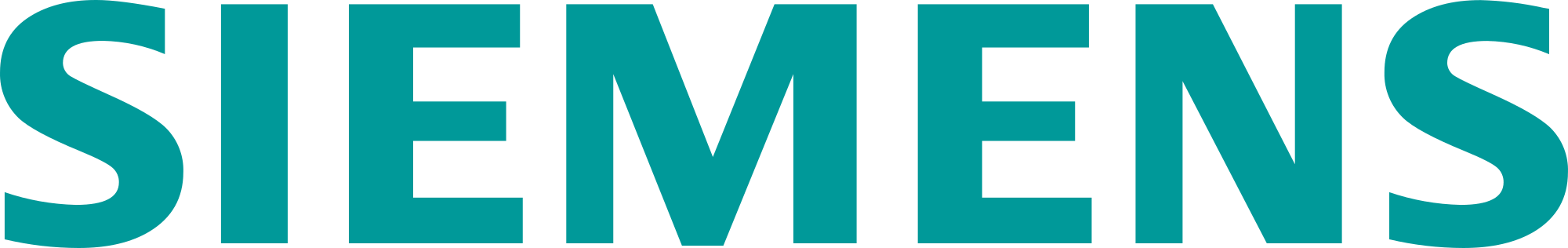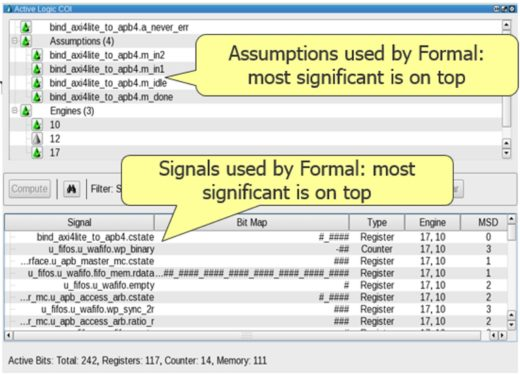# How to Reduce the Complexity of Formal Analysis – Part 2 – Reducing the Complexity of Your Assumptions

When using formal property checking, users often encounter “inconclusive” results; meaning the combined complexity of the design, assertions, and assumptions is beyond the capacity of the tool to compute in one run. In Part 1 of this series we outlined the basic steps you can take to get the analysis to converge. In this post we will focus on how you can reduce the complexity of your “assumption” properties to make them easier for the formal engines to digest and reach a solution.

As shown below, the assumptions in Questa PropCheck’s “Active Logic COI” tab are ordered in such a way that the assumption that affects the analysis the most is on top of the list.Figure 1: Active Logic Cone Of Influence (COI) window in Questa PropCheck, with all of the “Assumption” properties shown in the upper half of the report. This list is ordered, with the most influential assumption listed at the top. The logic “pulled in” to the analysis by the assumption is shown in the lower half of the report.

Checking the assumptions starting from the top, we can try removing some, re-coding them, or consider adding new ones. Specifically, since Questa PropCheck can clearly show the effect of an assumption on the logic cone of an assertion, the user can see if a particular assumption drags in extra design signals that are not in the cone of influence of an assertion. If this is occurring, re-coding the assumption – or even removing it entirely — to get rid of unnecessary design signals can reduce the overall logic cone. This can substantially improve the performance of the analysis, and itself could be all you need to do to get the proof.

IMPORTANT: if you are coming from the constrained-random simulation world (and who isn’t?), please do take a second to internalize this somewhat counterintuitive point about “assumptions” – a/k/a constraints — in the formal world: LESS is MORE!  In constrained-random simulation, quite often adding more constraints can help the constraint solver converge; or at a minimum, the extra constraints don’t have much performance impact whether or not they are actually applicable to a given test. However, if you put an assumption property into a formal analysis that is actually irrelevant to the area of logic/functionality you are looking to verify, formal will dutifully consider the logic touched by the unneeded assumption property and put clock cycles and memory on the case – unwittingly wasting compute resources and time.

Assumptions can also cause trouble when they allow too many value combinations, with the resulting complexity negatively impacting the formal run. In this case, the first step is to limit the values to a smaller scope. For example, consider this example with an address range of 0 to 256:

```addr_range: assume property (@(posedge clk)
address >= 0 && address <= 256 );

```

We can reduce the address range to reduce the complexity – start by cutting the range in half, and iterate as necessary.

Finally, a common way to limit the input changes is to set input to symbolic constant. The assume property is as follows.

```symbolic_sigA: assume property (@(posedge clk
##1 sigA == \$past(sigA));

```

At the first cycle, ‘sigA’ is not constrained, and formal considers all values. After the first cycle, ‘sigA’ retains its value. This assumption effectively makes “sigA” to be any constant value — called symbolic constant. The trick is that setting the data path to symbolic constant can help performance if the assertion doesn’t depend on the actual value of data.

What if you have tried all the above, and you can see the analysis getting a little farther down the road; but it’s still coming up inclusive?  In the next post we will show how to “decompose” your assertions to make it easier for the formal algorithms to reach a solution, without impacting the accuracy of the results.

Until then, may your state spaces be shallow, and your analyses be exhaustive!

Jin Hou and Joe Hupcey III
for the Questa Formal Team